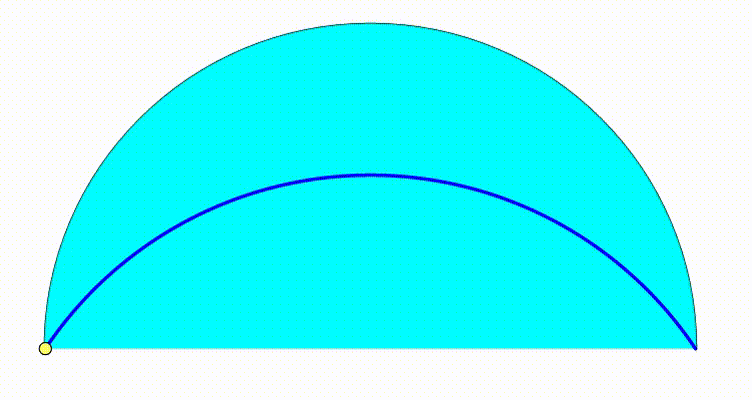# Dynamic Geometry: P95The diagram shows a black semicircle with radius $1$. The cyan and green semicircle are growing and shrinking freely on the black semicirle's diameter. The purple circle is internally tangent to the black semicircle and tangent to both green and cyan semicircle. The red and orange circles are internally tangent to the black semicircle and tangent to the purple circle and to one of the bottom semicircles. The tangency points between the orange circle, the red circles and the bottom semicircles trace a locus (blue curve). The area bounded by the blue curve and the black semicircle's diameter can be expressed as:

$\frac{p}{q} \cdot \tan^{-1}\left(\frac{m}{n}\right)-\frac{n}{m}$

where $p$, $q$, $m$ and $n$ are positive integers. $p$ and $q$ are coprime so are $m$ and $n$. Find $\sqrt{p+q+m+n}$.

×